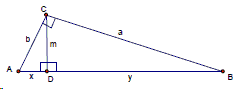# Lipogrammatic Proof of the Pythagorean Theorem

Learning a language means in part mastering its grammar and following its syntax rules. Writing grammatically is required of kids in school and adults at work, even if writing is not their occupation. Perhaps surprisingly, there are exceptions in the writing profession. A stark example is e e cummings who is known to have experimented writing prose and poetry with no capital letters or periods. Other professionals invent extra rules to follow in their work. Members of the French literary group Oulipo [Gardner, Ch 6,7] employed multiple devices to help generate ideas and enliven their writings. One of these is lipogram which is a manner (and the result) of writing that does not use a specific letter (or more.)

Mathematical language also has a grammar, and mathematicians follow various (often self-imposed) rules in their work. A well-known example is the restriction of the geometric constructions tool set to straightedge and compass. But why stop here? Additional constraints may help enliven any problem.

Most recently I received a communication from Michael Brozinsky who showed how to derive the ratio between the legs of right triangles with a congruent acute angle without mentioning their similarity. Based on this, Michael came up with a modified version of one of the proofs (Proof #6) of the Pythagorean theorem that eschewed mentioning hypotenuse until the very last.

Below I follow Michael Brozinsky's derivation.

### Lemma

Two right triangles $CAB$ and $FCE$ have $\angle CAB$ congruent to $\angle FCE$.Conclusion: their legs are in the same ratio, namely, $\frac{y}{x}=\frac{w}{z}$.

### Proof

Positioned as shown, the areas of triangles $CAB$ and $FCE$ and the rectangle BCED add up to the area of $\triangle AFD$:

$\frac{xy}{2} + \frac{zw}{2} + yz = \frac{(x+z)(y+w)}{2},$

which after simplification reduces to $yz=xw$. This is exactly $\frac{y}{x}=\frac{w}{z}$, as required. (Michael notes that this is also a nice way to show that the concept of slope is well defined without referring to similar triangles.)

Now for a proof of the Pythagorean Theorem. Drop the altitude from the right angle to the hypotenuse:There are three triangles $\triangle ABC$, $\triangle ACD$, and $CBD$ with congruent acute angles. From Lemma

$\frac{m}{x}=\frac{y}{m}=\frac{a}{b}$

and so

 (1) $AB=x+y=m\left(\frac{b}{a}+\frac{a}{b}\right).$

Now since the area of triangles $ADC$ and $DCB$ add up to the area of $\triangle ACB$ we have after multiplying by $2$ that

 (2) $mx+my=ab$

and so

 (3) $m=\frac{ab}{x+y}.$

Hence from (1) and (3), $x+y=\frac{ab}{x+y}\left(\frac{b}{a}+\frac{a}{b} \right)$ so that $(x+y)^{2}=a^{2}+b^{2}$ which is the Pythagorean identity.

### References

1. M. Gardner, Penrose Tiles to Trapdoor Ciphers, W. H. Freeman and Company, 1989